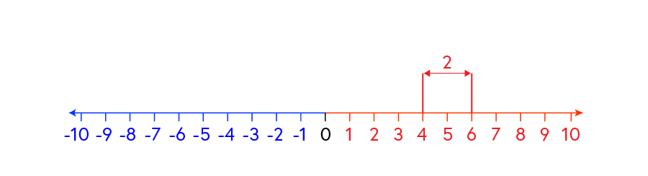## Want to keep learning?

This content is taken from the National STEM Learning Centre's online course, Maths Subject Knowledge: Understanding Numbers. Join the course to learn more.
3.5

# Subtraction with negative numbers: difference between

It is likely that ‘subtract’ is first met as the concept of ‘take away’. If you have ten sweets and I take away four sweets how many sweets have you left? This is very easy to model using concrete manipulatives: start with ten blocks, take away four blocks, how many blocks have you got left?

Taking away negative four blocks from your original ten blocks is very difficult to comprehend. Starting with negative ten blocks and taking away negative four blocks is equally challenging.

Another way to think of the operation ‘subtraction’ is as ‘the difference between’ two numbers rather than one number take away another.

## Consider the calculation 6 – 4.

First, emphasise that these are both positive numbers and the ‘-‘ sign is an operation: subtract. This can be achieved by rewriting the calculation as (+6) – (+4).

Language: rather than ‘take away’ refer to this as ‘the difference between’. For example: what is the difference between (+6) and (+4). Marking (+6) and (+4) on a number line we can see that the difference between the two numbers is 2.As 6 is larger than 4 we can say that 6 is 2 more than 4 giving a solution of (+2):

(+6) – (+4) = (+2) or 6 – 4 = 2.

We can also see that 4 is two less than 6. This tells us that the difference between 4 and 6 is -2 which can be written as (+4) – (+6) = (-2) or 4 – 6 = -2.

The advantage of approaching subtraction as the difference between two numbers on a number line is evident when considering subtracting negative numbers.

## Consider the calculation 6 – (-4)

What is the difference between 6 and (-4)? Mark +6 and -4 on a number line. Find the difference between the two numbers, in this case 10.

6 is 10 more than (-4) so the answer is (+10). 6 – (-4) = 10.

We can also see that the solution to the calculation (-4) – 6 is (-10). The difference between (-4) and 6 is 10, but (-4) is 10 less than 6 so the answer is (-10).